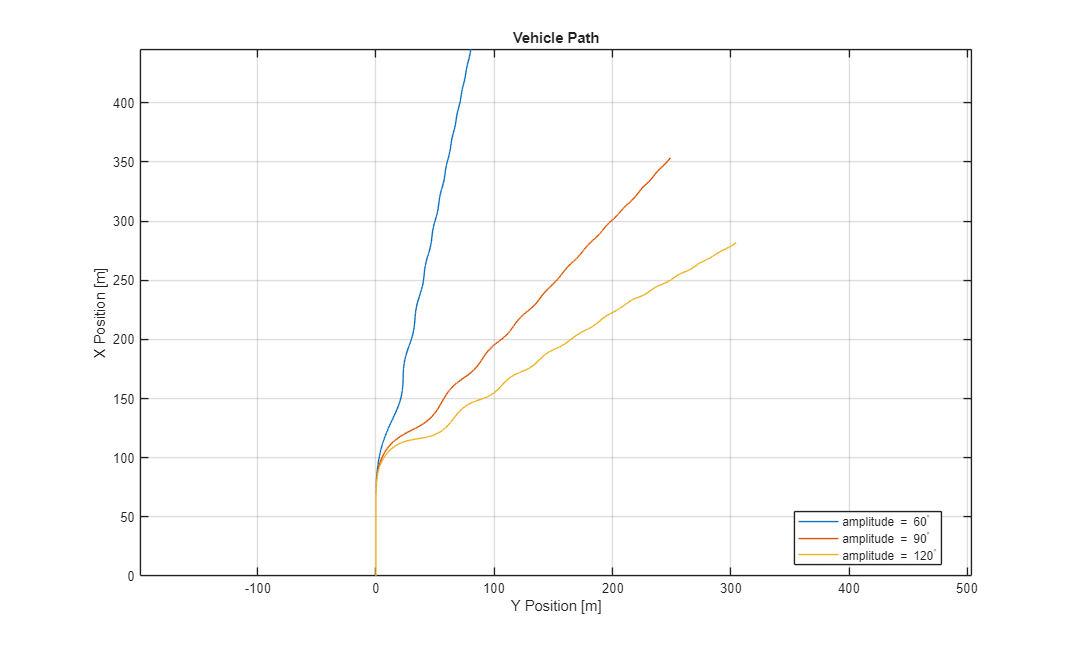# Frequency Response to Steering Angle Input

This example shows how to use the vehicle dynamics swept-sine steering reference application to analyze the dynamic steering response to steering inputs. Specifically, you can examine the vehicle frequency response and lateral acceleration when you run the maneuver with different sinusoidal wave steering amplitudes.

The swept-sine steering maneuver tests the vehicle frequency response to steering inputs. In the test, the driver:

• Accelerates until the vehicle hits a target velocity.

• Commands a sinusoidal steering wheel input.

• Linearly increase the frequency of the sinusoidal wave.

```helpersetupsss; ```### Run a Swept-Sine Steering Maneuver

1. Open the Swept Sine Reference Generator block. By default, the maneuver is set with these parameters:

• Longitudinal velocity setpoint — 30 mph

• Steering amplitude — 90 deg

• Final frequency — 0.7 Hz

2. In the Visualization subsystem, open the 3D Engine block. By default, the 3D Engine parameter is set to Disabled. For the 3D visualization engine platform requirements and hardware recommendations, see the Unreal Engine Simulation Environment Requirements and Limitations.

3. Run the maneuver with the default settings. As the simulation runs, view the vehicle information.

```mdl = 'SSSReferenceApplication'; sim(mdl); ```
```### Starting serial model reference simulation build. ### Model reference simulation target for Driveline is up to date. ### Model reference simulation target for PassVeh14DOF is up to date. ### Model reference simulation target for SiMappedEngineV is up to date. Build Summary 0 of 3 models built (3 models already up to date) Build duration: 0h 1m 31.723s ```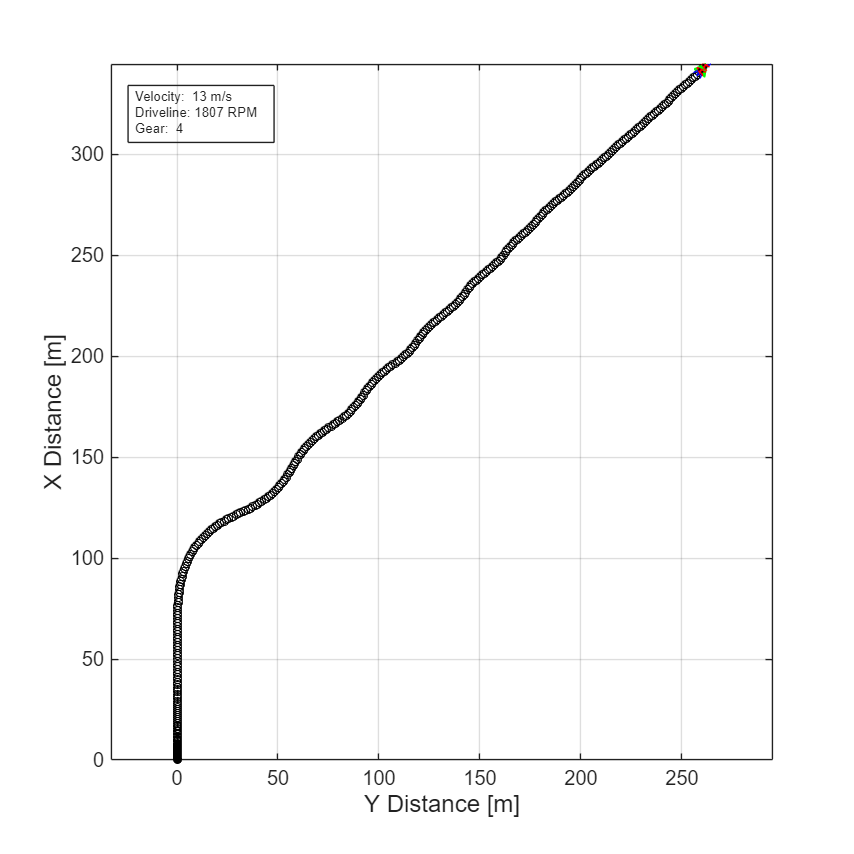• In the Vehicle Position window, view the vehicle longitudinal distance as a function of the lateral distance. The yellow line is the yaw rate. The blue line is the steering angle.

• In the Visualization subsystem, open the Yaw Rate and Steer Scope block to display the yaw rate and steering angle versus time.### Sweep Steering

Run the reference application with three different sinusoidal wave steering amplitudes.

1. In the swept-sine steering reference application model SSSReferenceApplication, open the Swept Sine Reference Generator block. The Steering amplitude, theta_hw block parameter sets the amplitude. By default, the amplitude is 90 deg.

2. Enable signal logging for the velocity, lane, and ISO signals. You can use the Simulink® editor or, alternatively, these MATLAB® commands. Save the model.

• Enable signal logging for the Lane Change Reference Generator outport Lane signal.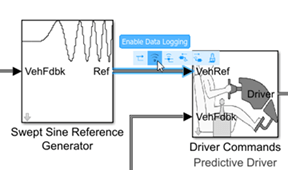```mdl = 'SSSReferenceApplication'; open_system(mdl); ph=get_param('SSSReferenceApplication/Swept Sine Reference Generator','PortHandles'); set_param(ph.Outport(1),'DataLogging','on'); ```
• Enable signal logging for the Passenger Vehicle block outport signal.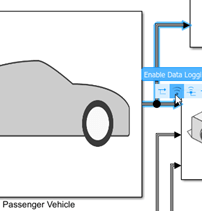```ph=get_param('SSSReferenceApplication/Passenger Vehicle','PortHandles'); set_param(ph.Outport(1),'DataLogging','on'); ```
• In the Visualization subsystem, enable signal logging for the ISO block.```set_param([mdl '/Visualization/ISO 15037-1:2006'],'Measurement','Enable'); ```

3. Set up a steering amplitude vector, `amp`, that you want to investigate. For example, at the command line, type:

```amp = [60, 90, 120]; numExperiments = length(amp); ```

4. Create an array of simulation inputs that set the Swept Sine Reference Generator block parameter Steering amplitude, theta_hw equal to `amp`.

```for idx = numExperiments:-1:1 in(idx) = Simulink.SimulationInput(mdl); in(idx) = in(idx).setBlockParameter([mdl '/Swept Sine Reference Generator'],... 'theta_hw',num2str(amp(idx))); end ```

5. Save the model and run the simulations. If available, use parallel computing.

```save_system(mdl) tic; simout = parsim(in,'ShowSimulationManager','on'); toc; ```
```[03-Mar-2023 13:41:52] Checking for availability of parallel pool... Starting parallel pool (parpool) using the 'Processes' profile ... Connected to parallel pool with 6 workers. [03-Mar-2023 13:42:57] Starting Simulink on parallel workers... [03-Mar-2023 13:43:23] Loading project on parallel workers... [03-Mar-2023 13:43:23] Configuring simulation cache folder on parallel workers... [03-Mar-2023 13:43:37] Loading model on parallel workers... [03-Mar-2023 13:44:21] Running simulations... [03-Mar-2023 13:48:03] Completed 1 of 3 simulation runs [03-Mar-2023 13:48:04] Completed 2 of 3 simulation runs [03-Mar-2023 13:48:04] Completed 3 of 3 simulation runs [03-Mar-2023 13:48:04] Cleaning up parallel workers... Elapsed time is 396.449678 seconds. ```

6. After the simulations complete, close the Simulation Data Inspector windows.

### Use Simulation Data Inspector to Analyze Results

Use the Simulation Data Inspector to examine the results. You can use the UI or, alternatively, command-line functions.

1. Open the Simulation Data Inspector. On the Simulink Toolstrip, on the Simulation tab, under Review Results, click Data Inspector.

• In the Simulation Data Inspector, select Import.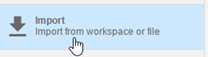• In the Import dialog box, clear `logsout`. Select `simout(1)`, `simout(2)`, and `simout(3)`. Select Import.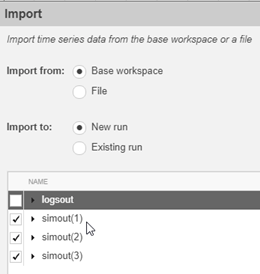• Use the Simulation Data Inspector to examine the results.

2. Alternatively, use these MATLAB commands to plot data for each run. For example, use these commands to plot the lateral position, steering wheel angle, and lateral acceleration. The results are similar to these plots, which show the results for each run.

```for idx = 1:numExperiments % Create sdi run object simoutRun(idx)=Simulink.sdi.Run.create; simoutRun(idx).Name=['Amplitude = ', num2str(amp(idx))]; add(simoutRun(idx),'vars',simout(idx)); end sigcolor=[0 1 0;0 0 1;1 0 1]; for idx = 1:numExperiments % Extract the lateral acceleration, position, and steering ysignal(idx)=getSignalsByName(simoutRun(idx), 'Passenger Vehicle:1.Body.InertFrm.Cg.Disp.Y'); ysignal(idx).LineColor =sigcolor((idx),:); ssignal(idx)=getSignalsByName(simoutRun(idx), 'Steering-wheel angle'); ssignal(idx).LineColor =sigcolor((idx),:); asignal(idx)=getSignalsByName(simoutRun(idx), 'Lateral acceleration'); asignal(idx).LineColor =sigcolor((idx),:); end Simulink.sdi.view Simulink.sdi.setSubPlotLayout(3,1); for idx = 1:numExperiments % Plot the lateral position, steering angle, and lateral acceleration plotOnSubPlot(ysignal(idx),1,1,true); plotOnSubPlot(ssignal(idx),2,1,true); plotOnSubPlot(asignal(idx),3,1,true); end ```

The results are similar to these plots, which indicate that the greatest lateral acceleration occurs when the steering amplitude is `120` deg.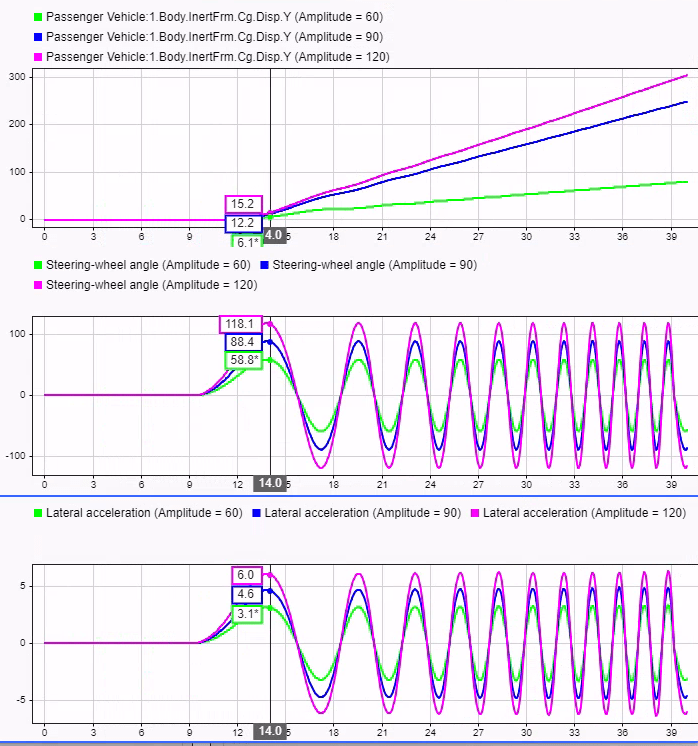### Further Analysis

To explore the results further, use these commands to extract the lateral acceleration, steering angle, and vehicle trajectory from the `simout` object.

1. Extract the lateral acceleration and steering angle. Plot the data. The results are similar to this plot.

```figure for idx = 1:numExperiments % Extract Data log = get(simout(idx),'logsout'); sa=log.get('Steering-wheel angle').Values; ay=log.get('Lateral acceleration').Values; legend_labels{idx} = ['amplitude = ', num2str(amp(idx)), '^{\circ}']; % Plot steering angle vs. lateral acceleration plot(sa.Data,ay.Data) hold on end % Add labels to the plots legend(legend_labels, 'Location', 'best'); title('Lateral Acceleration') xlabel('Steering Angle [deg]') ylabel('Acceleration [m/s^2]') grid on ```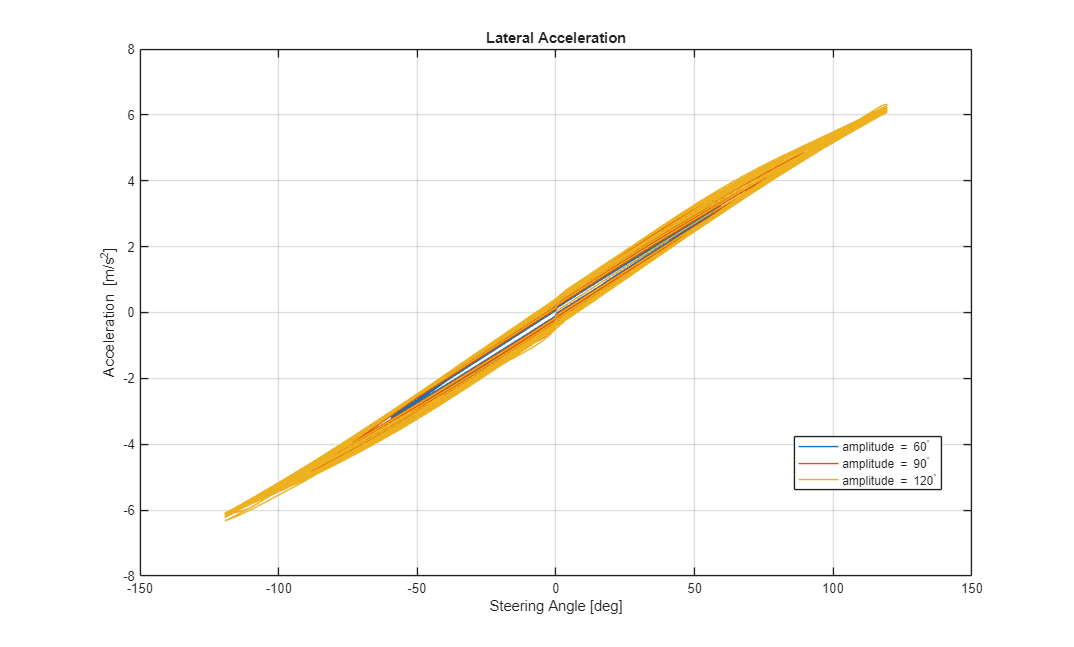2. Extract the vehicle path. Plot the data. The results are similar to this plot.

```figure for idx = 1:numExperiments % Extract Data log = get(simout(idx),'logsout'); xValues = getSignalsByName(simoutRun(idx), 'Passenger Vehicle:1.Body.InertFrm.Cg.Disp.X').Values; yValues = getSignalsByName(simoutRun(idx), 'Passenger Vehicle:1.Body.InertFrm.Cg.Disp.Y').Values; x = xValues.Data; y = yValues.Data; legend_labels{idx} = ['amplitude = ', num2str(amp(idx)), '^{\circ}']; % Plot vehicle location axis('equal') plot(y,x) hold on end % Add labels to the plots legend(legend_labels, 'Location', 'best'); title('Vehicle Path') xlabel('Y Position [m]') ylabel('X Position [m]') grid on ```